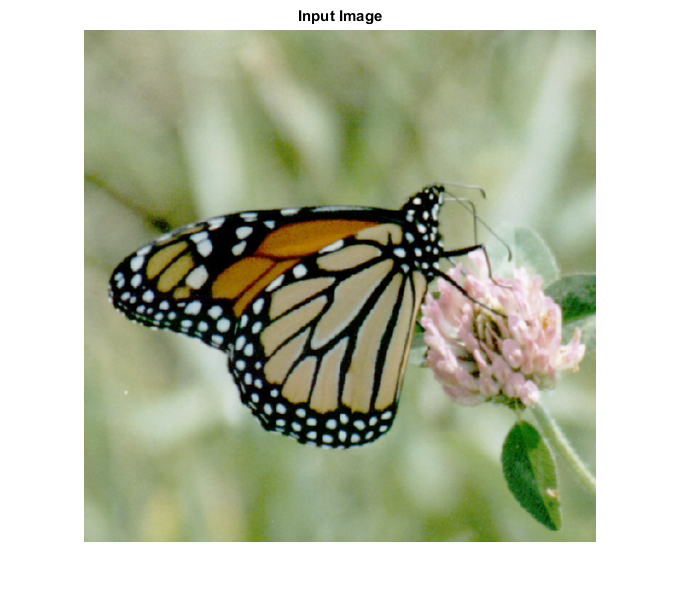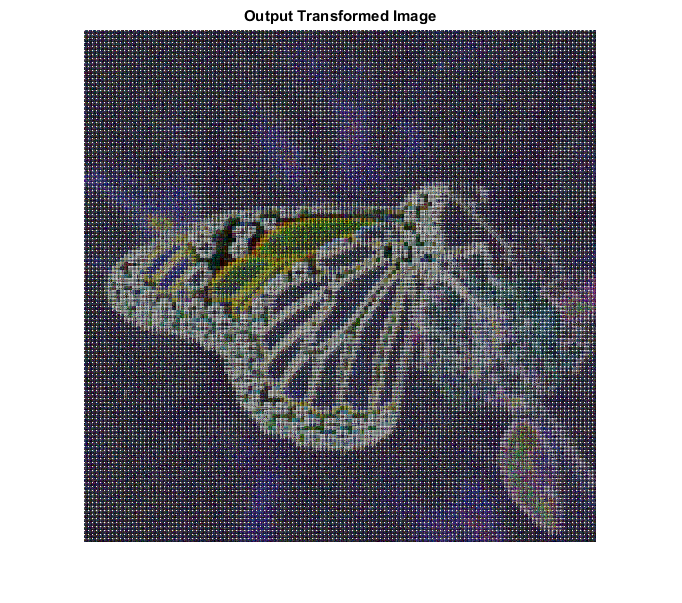## SYNTAX

`[ spect ] = walhad_cvip( input_img, block_size )`

Input Parameters Include :

• input_img - The orignial image which can be grayscale or RGB.
• block_size - Should be a power of 2 or [ ]. If empty, then functions finds the nearest power of 2 and zero pad the image,to that block size.Any other value smaller than image size will partiotion the image to windows of that block size and WHT will be calculated separately in each window.

Output Parameters Include :

• Spect - The Walsh/Hadamard transform of the input image.

## DESCRIPTION

This function performs the Walsh/Hadamard transform of an input image. The Walsh/Hadamard transform uses rectangular function as the basis vectors. These vectors consist only of '1's and '-1's. This transform is easy to compute than Fourier and Discrete Cosine transforms. The function performs the WHT transform on the input image and zero pads the spectrum to the size of the block_size specified by the user.

## REFERENCE

1. Scott E Umbaugh. DIGITAL IMAGE PROCESSING AND ANALYSIS: Applications with MATLAB and CVIPtools, 3rd Edition.

## EXAMPLE

```% Read Image

% Block size

block_size = 4;

% call function

% Display input image

figure;imshow(input_img);title('Input Image');

% Display output image

figure;imshow(remap_cvip(log(1+abs(spect))),[]);title('Output Transformed Image');
```